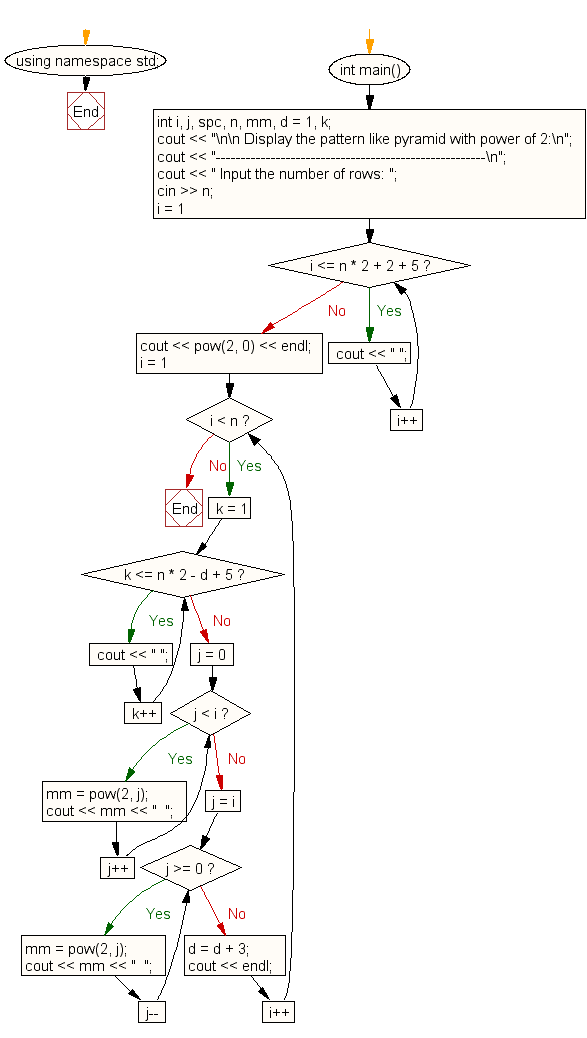﻿ C++ Exercises: Display the pattern power of 2, triangle - w3resource# C++ Exercises: Display the pattern power of 2, triangle

## C++ For Loop: Exercise-54 with Solution

Write a program in C++ to display the pattern power of 2, triangle.

Sample Solution:-

C++ Code :

``````#include <iostream>
#include <math.h>
using namespace std;

int main()
{
int i, j, spc, n, mm, d = 1, k;
cout << "\n\n Display the pattern like pyramid with power of 2:\n";
cout << "------------------------------------------------------\n";
cout << " Input the number of rows: ";
cin >> n;
//----------- space for first line ----------------------
for (i = 1; i <= n * 2 + 2 + 5; i++) //extra 5 spaces is the margin from left
cout << " ";
cout << pow(2, 0) << endl;
for (i = 1; i < n; i++)
{
//----------- printing spaces from 2nd line to end -------
for (k = 1; k <= n * 2 - d + 5; k++)
{
cout << " ";
}
//----------- print upto middle ----------------
for (j = 0; j < i; j++)
{
mm = pow(2, j);
cout << mm << "  "; //print 2 spaces
}
//------------- print after middle to end -------
for (j = i; j >= 0; j--)
{
mm = pow(2, j);
cout << mm << "  "; //print 2 spaces
}
d = d + 3;
cout << endl;
}
}
``````

Sample Output:

```Display the pattern like pyramid with power of 2:
------------------------------------------------------
Input the number of rows:
1
1  2  1
1  2  4  2  1
1  2  4  8  4  2  1
1  2  4  8  16  8  4  2  1
```

Flowchart:C++ Code Editor:

Contribute your code and comments through Disqus.

What is the difficulty level of this exercise?

﻿

## C++ Programming: Tips of the Day

What is a smart pointer and when should I use one?

This answer is rather old, and so describes what was 'good' at the time, which was smart pointers provided by the Boost library. Since C++11, the standard library has provided sufficient smart pointers types, and so you should favour the use of std::unique_ptr, std::shared_ptr and std::weak_ptr.

There was also std::auto_ptr. It was very much like a scoped pointer, except that it also had the "special" dangerous ability to be copied - which also unexpectedly transfers ownership.

It was deprecated in C++11 and removed in C++17, so you shouldn't use it.

```std::auto_ptr<MyObject> p1 (new MyObject());
std::auto_ptr<MyObject> p2 = p1; // Copy and transfer ownership.
// p1 gets set to empty!
p2->DoSomething(); // Works.
p1->DoSomething(); // Oh oh. Hopefully raises some NULL pointer exception.
```

Ref : https://bit.ly/3mc9GHE